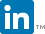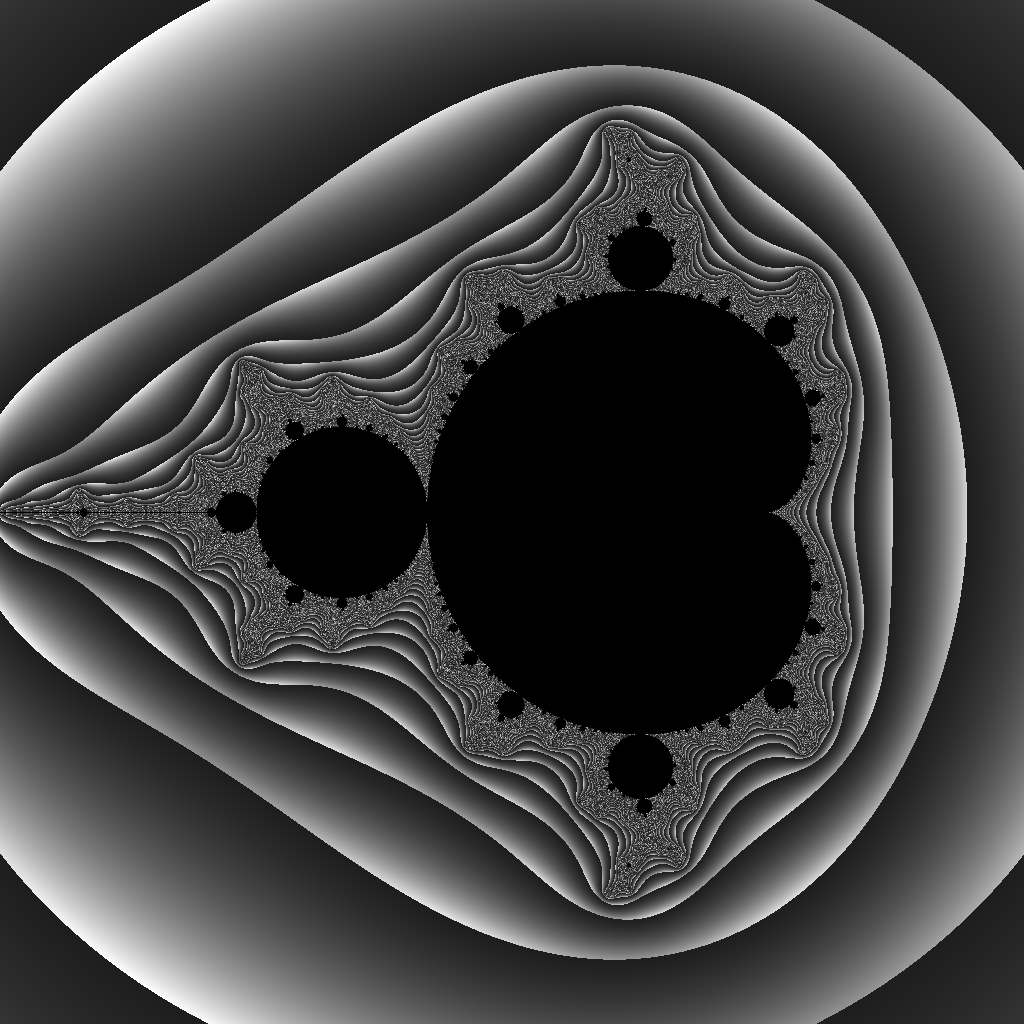# wee codes## projects | about | hobbies

Welcome to the personal project website for Jamie Blondin. Here, you’ll find several projects Jamie has been working on. For more of his work, visit his GitHub profile.

## agnes

A data wrangling library written in Rust. `agnes` is a data preprocessing library which allows library users to create robust, efficient, readable programs that load, aggregate, filter, and transform data sources.

The following code is taken from the `agnes` guide, which provides a demonstration of some of the basic data loading, joining, filtering, and transformation functionality `agnes` currently supports.

``````#[macro_use]
extern crate agnes;

tablespace![
table gdp {
CountryName: String,
CountryCode: String,
Gdp2015: f64,
}
];

fn main() {
let gdp_spec = spec![
fieldname gdp::CountryName = "Country Name";
fieldname gdp::CountryCode = "Country Code";
fieldname gdp::Gdp2015 = "2015";
];

// load the GDP CSV file from a URI
"https://wee.codes/data/gdp.csv",
gdp_spec

println!("{}", gdp_view);
}
``````
``````\$ ./my_gdp_loading_app
CountryName                                          | CountryCode | Gdp2015
------------------------------------------------------+-------------+------------------
Aruba                                                | ABW         | NA
Afghanistan                                          | AFG         | 19215562178.9798
Angola                                               | AGO         | 102962245246.708
Albania                                              | ALB         | 11335264966.561
Andorra                                              | AND         | 2811489408.89431
Arab World                                           | ARB         | 2563301730344.97
United Arab Emirates                                 | ARE         | 357949199754.935
Argentina                                            | ARG         | 584711485367.267
...
``````

`agnes` is currently in development, with much more planned for its future.

## matrix

A matrix library written in Rust. Supports basic addition, subtraction, and multiplication operations, solving systems of equations (including underdetermined and overdetermined), decompositions, and norms (both vector and matrix). Mainly built so I could learn my way around BLAS / LAPACK.

``````// get a positive semi-definite matrix from somewhere
let a = get_psd_matrix();

// it's supposed to be square!
let (m, n) = a.dims();
assert_eq!(m, n);

// get the cholesky decomposition
let chol_decomp = a.chol().expect("uh-oh, decomposition failed! \
are you sure the matrix is positive semi-definite?");

// extract the lower-triangular matrix
let l = chol_decomp.l();

// L * L'
let b = &l * l.t();

// frobenius norm should (hopefully) be small!!
assert!((a - b).matrix_norm(MatNorm::Frobenius) < 1.0e-12);
``````

## rhubarb

Rhubarb is a data visualization server package in the initial stages of design and development. Its goal is to provide a web application server framework in which users can specify how to load data (using, for instance, agnes), perform data preprocessing, and add this data into charts. Once run, the rhubarb app will serve charts – including interactive charts – to a web application running a rhubarb frontend which draws them using plotly.

## etl

A Rust data-loading library (and early precursor to agnes). This library provides functionality to apply a data file using a TOML configuration file and apply simple data transformations and filtering.

``````## data_config.toml

[[source_files]]
name = "source1.csv"
delimiter = ","
fields = [ { source_name = "a_text_field", field_type = "Text", add_to_frame = false },
{ source_name = "another_text_field", field_type = "Text", add_to_frame = false } ]

[[source_files]]
name = "source2.tsv"
delimiter = "\t"
fields = [ { source_name = "an_integer", field_type = "Signed" },
{ source_name = "another_integer", field_type = "Signed" },
{ source_name = "a_category", field_type = "Text" },
{ source_name = "an_unused_float", field_type = "Float", add_to_frame = false } ]

[[transforms]]
method = { action = "Concatenate",  separator = " & " }
source_fields = [ "a_text_field", "another_text_field" ]
target_name = "a_new_text_field"

[[transforms]]
source_fields = [ "a_category" ]
target_name = "category_mapped_to_integers"

[transforms.method]
action = "Map"
default_value = "-1"
map = { "first_category" = "0", "second_category" = "1" }
``````
``````let data_path = PathBuf::from(file!()).parent().unwrap().join("data_config.toml");

let mut fieldnames = df.fieldnames();
fieldnames.sort();
assert_eq!(fieldnames, ["a_category", "a_new_text_field", "an_integer", "another_integer"
"category_mapped_to_integers"]);
``````

This project is not currently under development, as much of its functionality has been (or soon will be) migrated over to agnes. However, I may revisit this project in the future, as a configuration-file-based data loading tool may still be useful (and could easily leverage `agnes`’s functionality).

## piske

A domain-specific programming language designed for the creation of generative art with concise, easy-to-understand syntax and semantics. The current implentation of piske includes both an intepreter and a transpiler into Rust (the lanague upon which it’s built).

Piske is currently in an alpha state, with many more features planned.

``````let height = 1024;
let width = 1024;
set_image_dims(height, width);

let camera_center = -0.5 + 0i;
let camera_size = 3 + 3i;

let threshold = 10;
let num_max_iters = 1000;

iterate row = [0, height) {
iterate col = [0, width) {
let z = 0 + 0i;
let c = project(row, col, camera_center, camera_size);
let value = iterate over [0, num_max_iters) {
z = z * z + c;
let escape_value = re(z * z`);
if escape_value > threshold {
break escape_value;
}
0.0
};
set_pixel_data(row, col, value);
}
}

write("output.png");
``````## sindra

Sindra is a library for developing programming languages. As I continue work in various programming languages (e.g. Piske), I plan on moving more common functionality into this library.